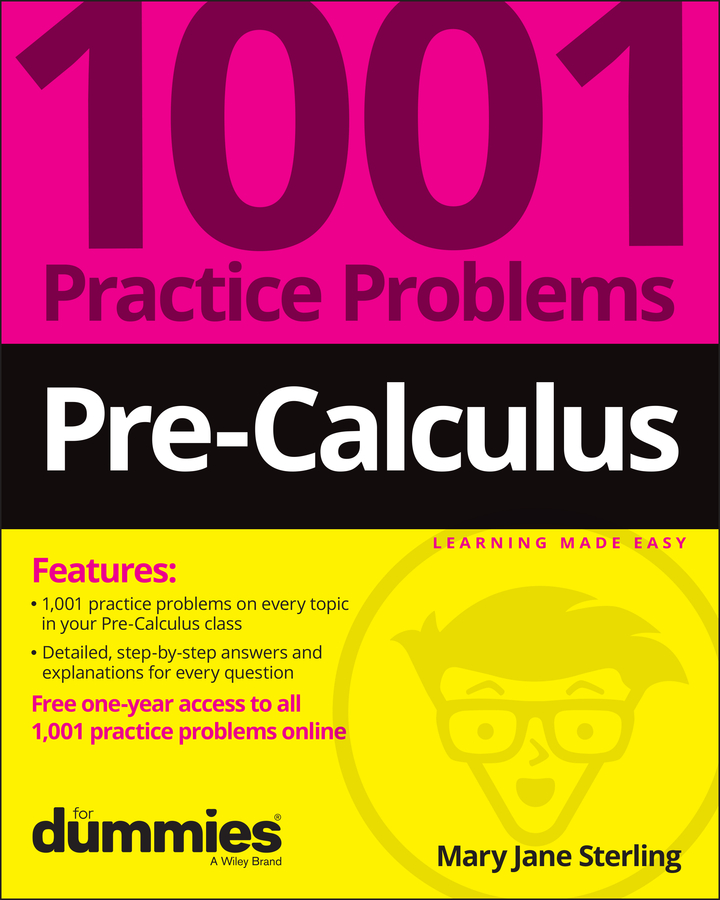##### Pre-Calculus: 1001 Practice Problems For Dummies (+ Free Online Practice)If you know how many total roots a polynomial has, you can use a pretty cool theorem called Descartes’s rule of signs to count how many roots are real numbers (both positive and negative) and how many are imaginary. You see, the same man who pretty much invented graphing, Descartes, also came up with a way to figure out how many times a polynomial can possible cross the x-axis — in other words, how many real roots it can possibly have. All you have to be able to do is count!

The terms solutions/zeros/roots are synonymous because they all represent where the graph of a polynomial intersects the x-axis. The roots that are found when the graph meets with the x-axis are called real roots; you can see them and deal with them as real numbers in the real world. Also, because they cross the x-axis, some roots may be negative roots (which means they intersect the negative x-axis), and some may be positive roots (which intersect the positive x-axis).

Here’s how Descartes’s rule of signs can give you the numbers of possible real roots, both positive and negative:

• Positive real roots. For the number of positive real roots, look at the polynomial, written in descending order, and count how many times the sign changes from term to term. This value represents the maximum number of positive roots in the polynomial. For example, in the polynomial f(x) = 2x4 – 9x3 – 21x2 + 88x + 48, you see two changes in sign (don’t forget to include the plus sign of the first term!) — from the first term (+2x4) to the second (-9x3) and from the third term (-21x2) to the fourth term (88x). That means this equation can have up to two positive solutions.

Descartes’s rule of signs says the number of positive roots is equal to changes in sign of f(x), or is less than that by an even number (so you keep subtracting 2 until you get either 1 or 0). Therefore, the previous f(x) may have 2 or 0 positive roots.

• Negative real roots. For the number of negative real roots, find f(–x) and count again. Because negative numbers raised to even powers are positive and negative numbers raised to odd powers are negative, this change affects only terms with odd powers. This step is the same as changing each term with an odd degree to its opposite sign and counting the sign changes again, which gives you the maximum number of negative roots. The example equation becomes f(–x) = 2x4 + 9x3 – 21x2 – 88x + 48, which changes signs twice. There can be, at most, two negative roots. However, similar to the rule for positive roots, the number of negative roots is equal to the changes in sign for f(–x), or must be less than that by an even number. Therefore, this example can have either 2 or 0 negative roots.